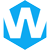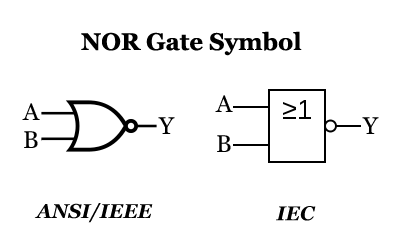# NOR Gate Simplifying Boolean Expression## Introduction to Boolean expressions

Boolean expressions are fundamental components of digital logic design. They represent logical relationships between variables, typically resulting in a binary output. These expressions are crucial for building efficient and reliable digital circuits. In this article, we will delve into the world of Boolean expressions and focus on one specific gate: the NOR gate.

## What is a NOR gate?

A NOR gate, short for “NOT OR” gate, is a logic gate that produces a high output only when all its inputs are low. It behaves as an inverted OR gate, meaning it outputs a low signal if any of its inputs are high. The NOR gate is represented by a symbol resembling an OR gate followed by a small circle at its output. This circle indicates the inversion of the output signal.

## NOR gate truth table and logic symbol

To understand the behavior of a NOR gate, let’s examine its truth table:

Input AInput BOutput
001
010
100
110

As seen in the truth table, the NOR gate outputs a high signal only when both inputs are low. If any input is high, the output becomes low. This behavior makes the NOR gate ideal for simplifying Boolean expressions and reducing the complexity of logic circuits.

The logic symbol for a NOR gate visually represents its operation. It consists of two or more inputs connected to the curved part of the OR gate symbol, and the output is indicated by a small circle at the end.

## Simplifying Boolean expressions using NOR gates

One of the key advantages of NOR gates is their ability to simplify complex Boolean expressions. By utilizing DeMorgan’s theorem, which states that the complement of an OR operation is equivalent to the AND operation of the complements, we can transform complex expressions into simpler forms.

For example, let’s consider the expression: A + B. To simplify this expression using a NOR gate, we can apply DeMorgan’s theorem. First, we take the complement of A and B, resulting in A’ and B’. Then, we connect A’ and B’ as inputs to a NOR gate. The output of this NOR gate will be the simplified form of the expression: (A + B)’.

This simplification technique allows us to reduce the number of gates required in a circuit, leading to improved efficiency and reduced complexity.

## DeMorgan's theorem and its application to NOR gates

DeMorgan’s theorem is a fundamental concept in digital logic design that provides a method for transforming complex expressions into simpler forms. It states that the complement of an AND operation is equivalent to the OR operation of the complements, and vice versa.

When applying DeMorgan’s theorem to NOR gates, we can simplify expressions by converting AND operations to NOR operations and vice versa. This allows us to optimize logic circuits and reduce the number of gates required.

For instance, let’s consider the expression: (A + B)’. By applying DeMorgan’s theorem, we can rewrite this expression as A’ · B’. Here, the OR operation has been transformed into an AND operation. This transformation is especially useful when designing circuits with NOR gates, as it enables us to utilize the simplicity and efficiency of NOR gates.

## Examples of simplifying Boolean expressions using NOR gates

To illustrate the simplification process using NOR gates, let’s explore a few examples:

### Example 1: Simplify the expression: A · B + C · D.

First, we take the complement of each input: A’, B’, C’, D’.
Then, we construct a NOR gate with inputs A’, B’, C’, and D’.
The output of this NOR gate will be the simplified form of the expression: (A · B + C · D)’.

### Example 2: Simplify the expression: A · (B + C)'.

We start by applying DeMorgan’s theorem to the expression within the parentheses: (B + C)’ becomes B’ · C’.
Then, we connect A, B’, and C’ as inputs to a NOR gate.
The output of this NOR gate will be the simplified form of the expression: A · (B + C)’.

These examples demonstrate how NOR gates can be effectively used to simplify Boolean expressions and reduce circuit complexity.

## Advantages of using NOR gates in logic design

Using NOR gates in logic design offers several advantages:

### Simplified circuitry:

NOR gates can simplify complex Boolean expressions, reducing the number of gates required in a circuit. This simplification leads to more efficient and compact designs.

### Cost-effective implementation:

NOR gates are relatively simple to implement, making them cost-effective for large-scale integration. Their simplicity also aids in troubleshooting and maintenance of digital circuits.

### Universal functionality:

NOR gates can be combined to create any other type of logic gate, such as AND, OR, and NOT gates. This universality makes NOR gates versatile components in digital circuit design.

### Noise immunity:

NOR gates exhibit excellent noise immunity due to their ability to reject any input noise. This characteristic ensures reliable operation in noisy environments.

## NOR gate applications in digital circuits

NOR gates find extensive applications in digital circuits due to their unique properties. Some common applications include:

### Memory units:

NOR gates are the building blocks of various memory units, such as SRAM (Static Random-Access Memory) and NOR flash memory. These memory units provide non-volatile storage in computers and other electronic devices.

### Programmable logic arrays (PLAs):

PLAs utilize NOR gates to implement complex logic functions. They offer flexibility in designing custom logic functions, making them widely used in digital systems.

### Digital counters:

NOR gates are essential components in digital counters, enabling the counting and sequencing of digital signals. These counters are commonly used in electronic devices like timers and frequency dividers.

### Logic gates and flip-flops:

NOR gates are used to build various logic gates, such as AND, OR, and XOR gates. They are also utilized in constructing flip-flops, which are crucial for storing and manipulating binary information.

## Other types of logic gates and their relation to NOR gates

While NOR gates have their unique advantages, it is essential to understand their relationship with other types of logic gates:

• AND gate: An AND gate can be constructed using a NOR gate followed by a NOT gate. The inputs of the NOR gate are connected to the inputs of the AND gate, and the output of the NOR gate is fed into the input of the NOT gate.
• OR gate: An OR gate can be implemented using a NOR gate followed by a NOT gate. The inputs of the NOR gate are connected to the inputs of the OR gate, and the output of the NOR gate is inverted using the NOT gate.
• NOT gate: A NOT gate, also known as an inverter, can be implemented using a NOR gate with both inputs tied together. The output of the NOR gate becomes the inverted form of the input signal.

Understanding the relationship between NOR gates and other logic gates allows for flexible circuit design and efficient utilization of different gate types.

## Conclusion

In conclusion, understanding the NOR gate is essential for simplifying Boolean expressions and designing efficient logic circuits. NOR gates provide a powerful tool for reducing circuit complexity and improving the overall performance of digital systems. By applying DeMorgan’s theorem, we can transform complex expressions into simpler forms, making use of the unique properties of NOR gates. Their versatility, cost-effectiveness, and noise immunity make NOR gates invaluable components in digital circuit design. So, embrace the NOR gate, and unlock the potential for efficient logic design.

#### Share:Aidan Taylor

I am Aidan Taylor and I have over 10 years of experience in the field of PCB Reverse Engineering, PCB design and IC Unlock.

## Recommended Post

### Need Help?

Don't hesitate - Get in touch today to find out how we can help take your project to the next level.

Scroll to Top## Start to Achieve Your PCB Project

Hello 👋
Can we help you? Chat with us on WhatsApp↓, or send an email to us: info@reversepcb.com